The least-squares method for approximation of data
using
Mathematica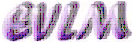Background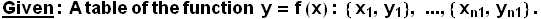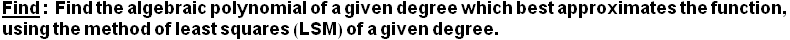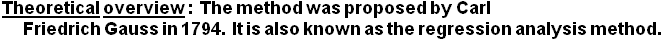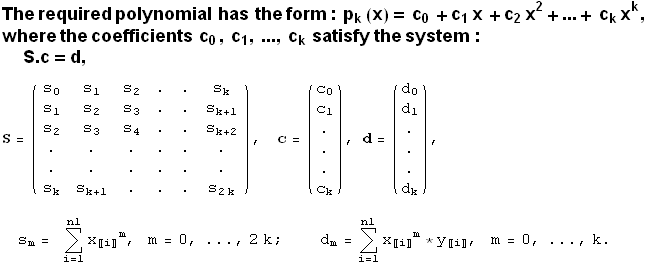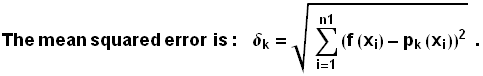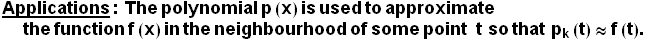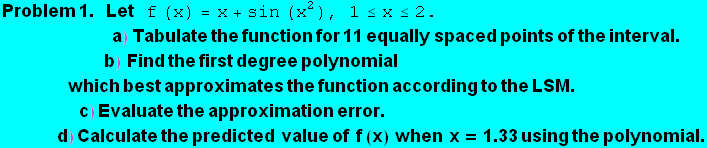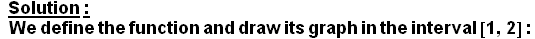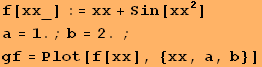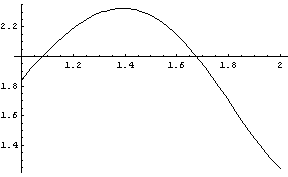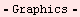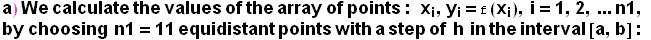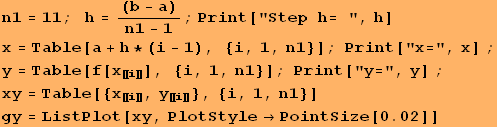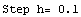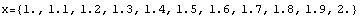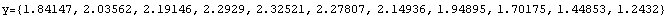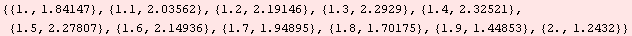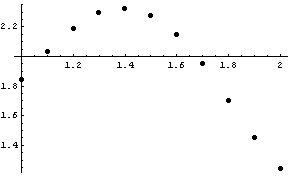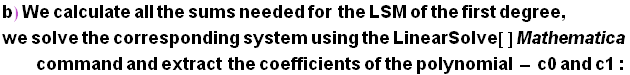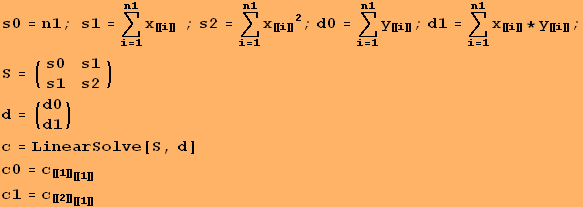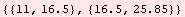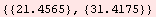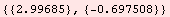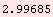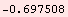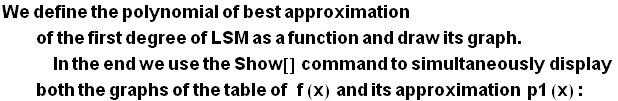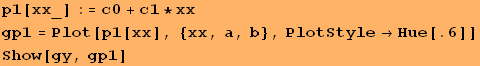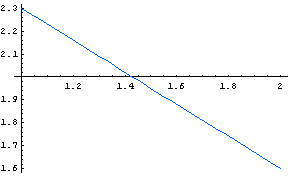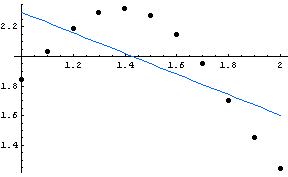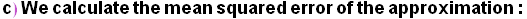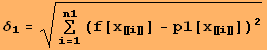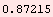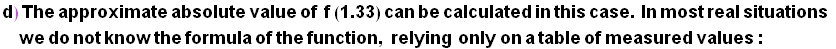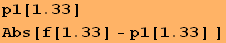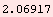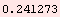Conclusion:   The obtained error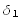is obviously large as can also be seen from the graphs of both functions. An approximation with a polynomial of a higher degree can be found.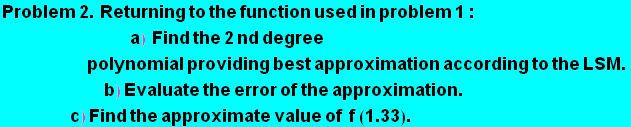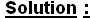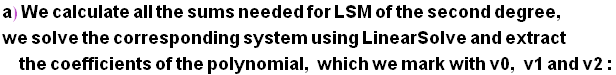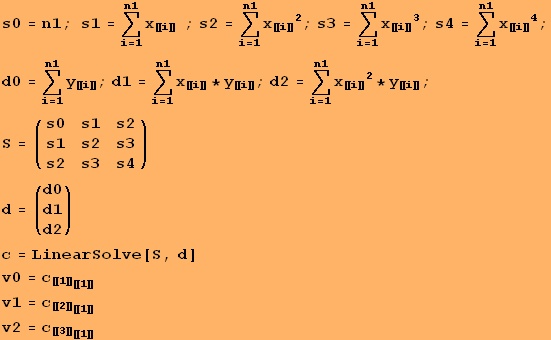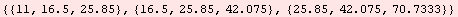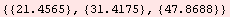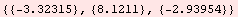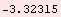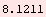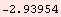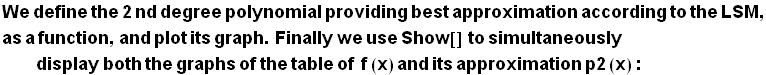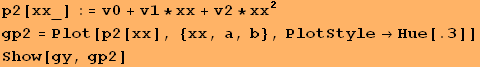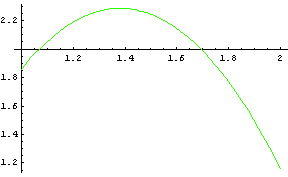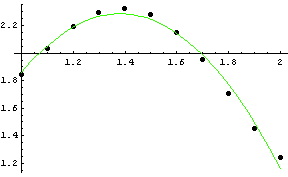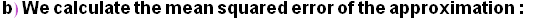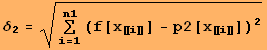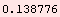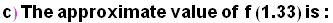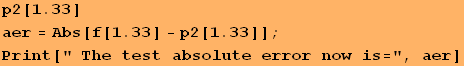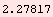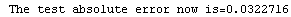Conclusion:   The derived errors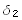and aer are obviously still large but are more acceptable than the former approximation (compare the graphs of the two functions).  An approximation with a polynomial of an even higher degree can be found.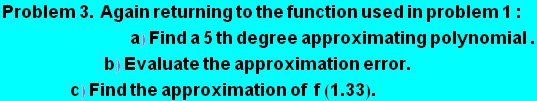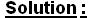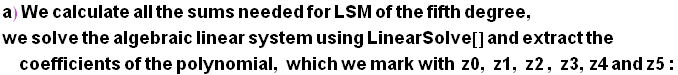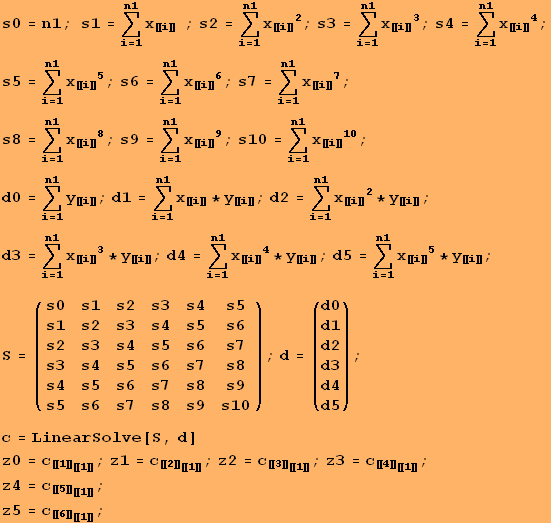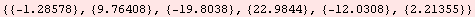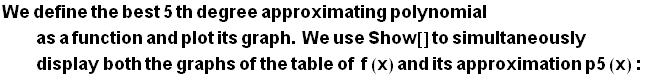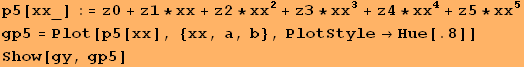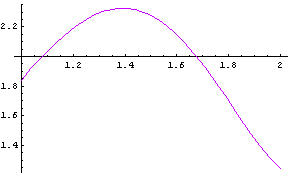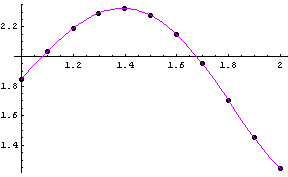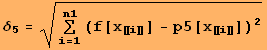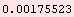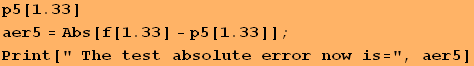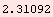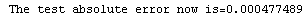Conclusion:   The derived error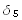and the test absolute error are now quite satisfactory.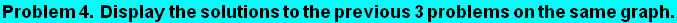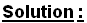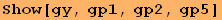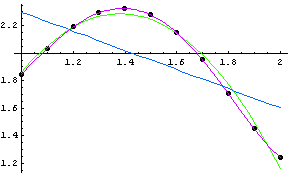Created by Mathematica  (May 22, 2008)